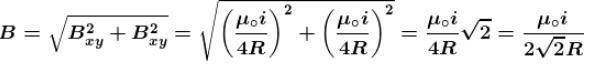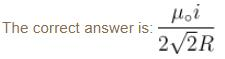Courses

# Magnetic Effect Of Current MCQ Level - 1

## 10 Questions MCQ Test Topic wise Tests for IIT JAM Physics | Magnetic Effect Of Current MCQ Level - 1

Description
This mock test of Magnetic Effect Of Current MCQ Level - 1 for Physics helps you for every Physics entrance exam. This contains 10 Multiple Choice Questions for Physics Magnetic Effect Of Current MCQ Level - 1 (mcq) to study with solutions a complete question bank. The solved questions answers in this Magnetic Effect Of Current MCQ Level - 1 quiz give you a good mix of easy questions and tough questions. Physics students definitely take this Magnetic Effect Of Current MCQ Level - 1 exercise for a better result in the exam. You can find other Magnetic Effect Of Current MCQ Level - 1 extra questions, long questions & short questions for Physics on EduRev as well by searching above.
QUESTION: 1

### A current i is flowing in a straight conductor of length L. The magnetic induction at a point distant L/4 from its centre will be :

Solution: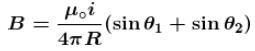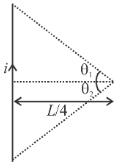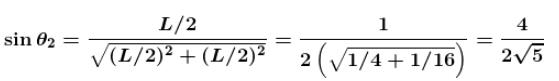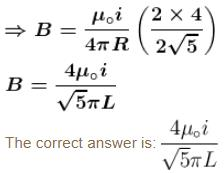QUESTION: 2

### A long solenoid carrying a current produce’s a magnetic field B along its axis. If the current is doubled acid the number of turns per cm  is halved, the new value of the magnetic field is :

Solution: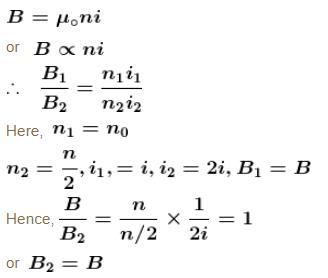QUESTION: 3

### For paramagnetic materials magnetic susceptibility is related with temperature as :

Solution:

For paramagnetic materials magnetic susceptibility is inversely proportional to the temperature i.e., proportional to  T–1.

QUESTION: 4

An electron and proton enter a uniform magnetic field perpendicular. Both have same kinetic energy. Which of the following is true?

Solution:

When a charged particle enters a magnetic field perpendicularly, it moves on a circular path. The required centripetal force is provided by magnetic force.

i.e. magnetic force = Centripetal force.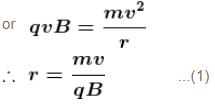Now kinetic energy of the particle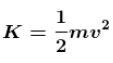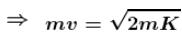Therefore, Eq. (1) become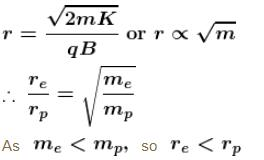Hence trajectory of proton is less curved.

The correct answer is: Trajectory of proton is less curved

QUESTION: 5

A charge of 10–6C is describing a circular path of radius 1cm making 5 revolution per second.  The magnetic induction field at the centre of the circle is :

Solution: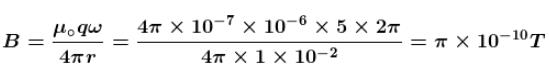The correct answer is: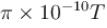QUESTION: 6

A charged particle enters a uniform magnetic field with velocity vector at an angle of 45° with the magnetic field. The pitch of the helical path followed by the particle is  p. The radius of the helix will be :

Solution: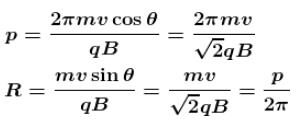The correct answer is: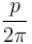QUESTION: 7

A charged particle (charge  q) is moving in a circle of radius R with uniform speed v. The associated magnetic moment µ is given by :

Solution:

As revolving charge is equivalent to a current, so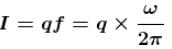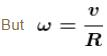where R is radius of circle and v is uniform speed of charged particle.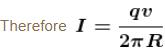Now, magnetic moment associated with charged particle is given by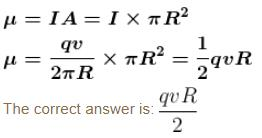QUESTION: 8

The magnetic induction at centre O  in the following figure will be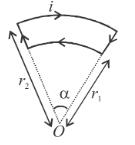Solution: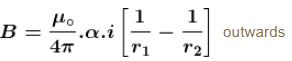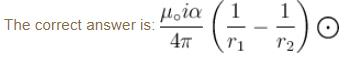QUESTION: 9

A current carrying wire is bent in the L shape as shown in figure. The length of both arms extend to infinity. Then, the magnetic field at O is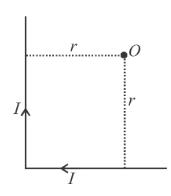Solution: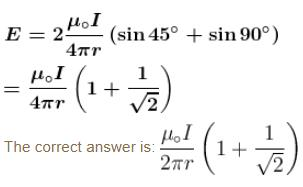QUESTION: 10

A current loop consists of two identical semicircular parts each of radius R, one lying in the x - y plane and the other in x - z plane. If the current in the loop is i. The resultant magnetic field due to the two semiconductor parts at their common centre is :

Solution:

The loop mentioned in the question must look like one as shown in the figure.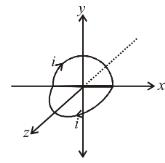Magnetic field at the centre due to semicircular loop lying in x-y plane.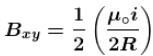in negative z direction.

Similarly field due to loop in x-z plane,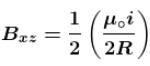in negative y direction.

∴ Magnetic of resultant magnetic field,